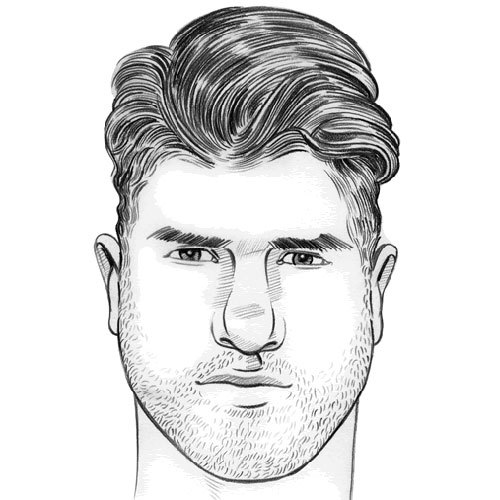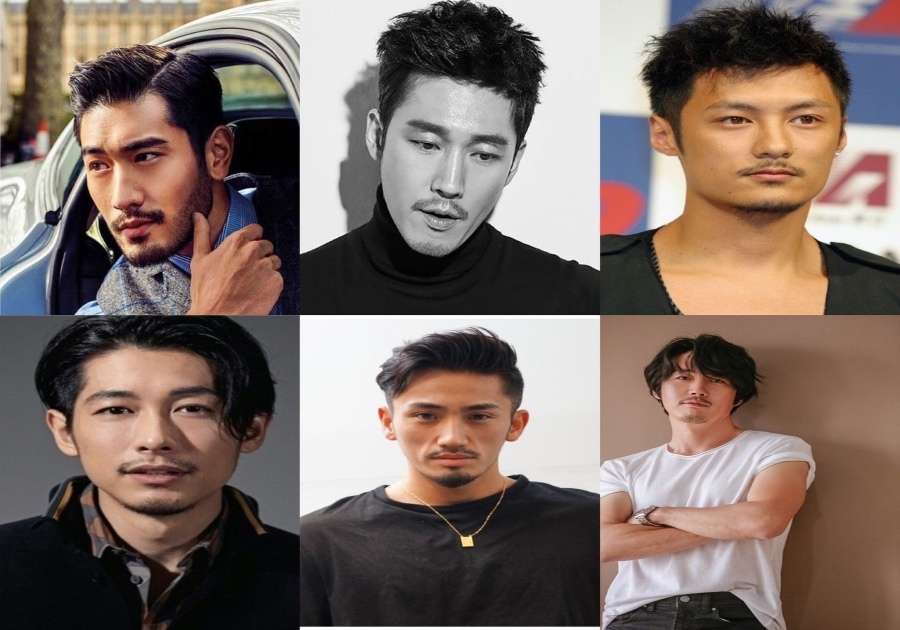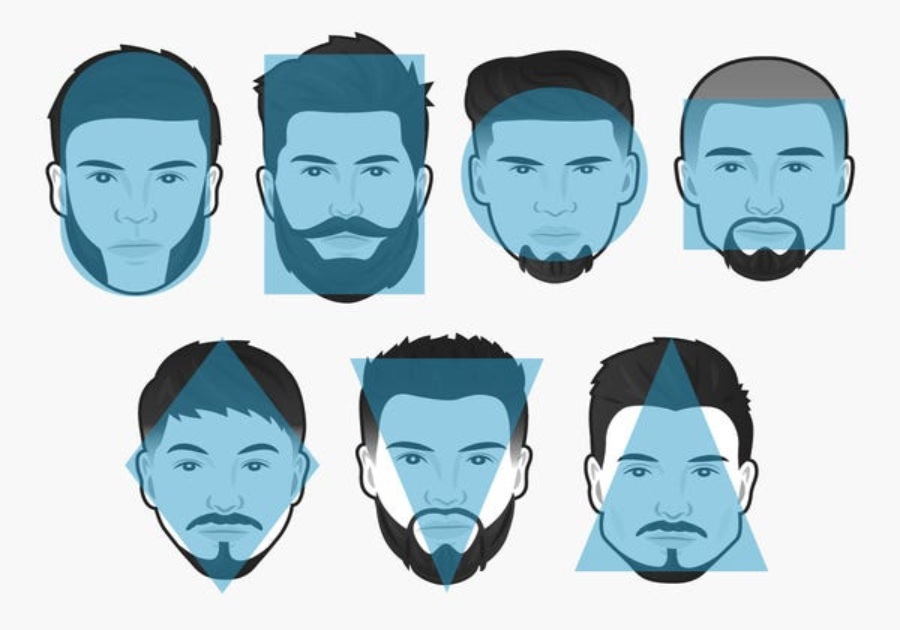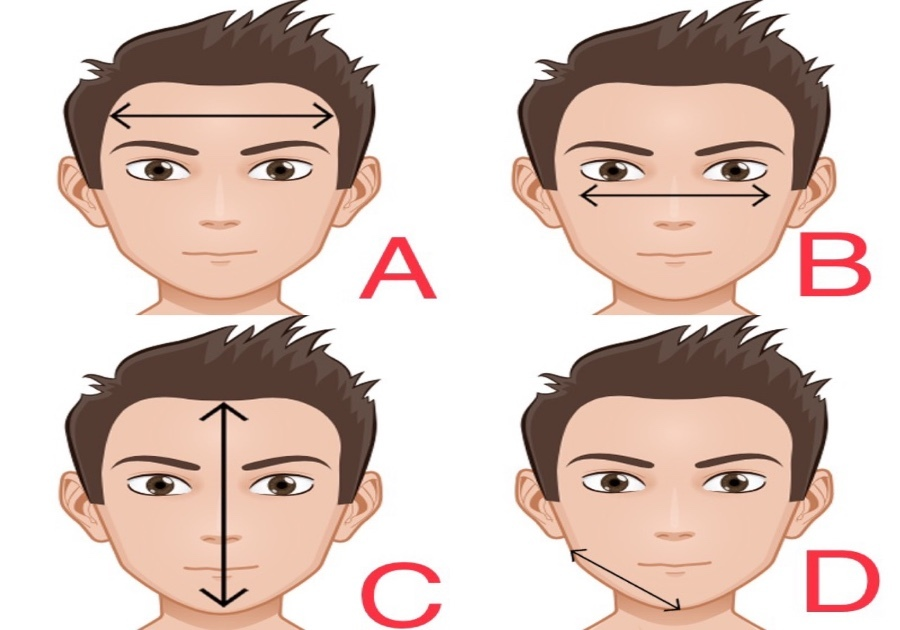Oval橢圓形臉：( C> B ) + ( A > D )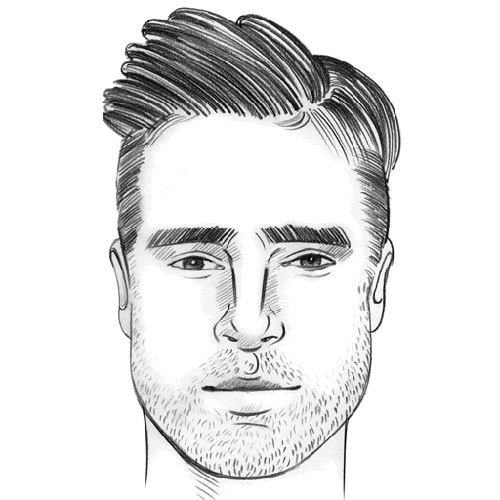Round圓形臉：C = B > A = D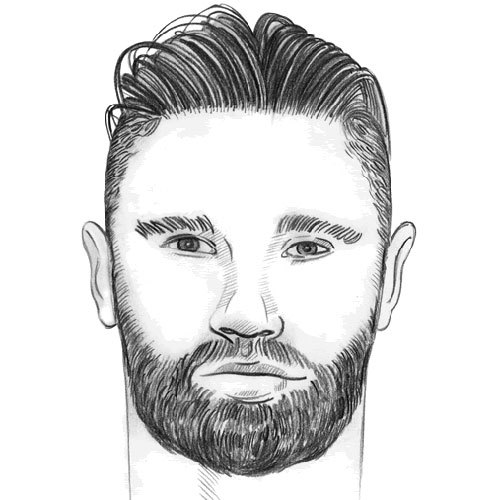Square方形臉：C = B = A = D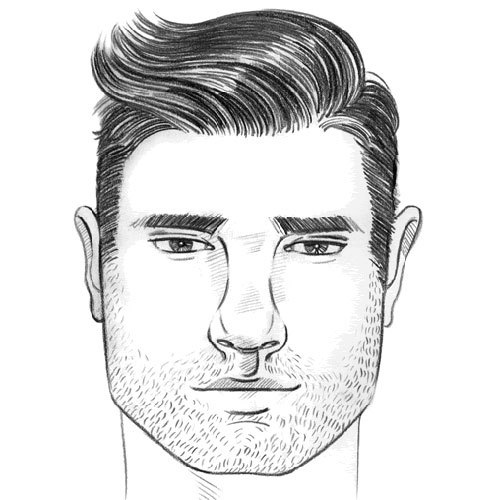Oblong長方形臉：C > B = A = D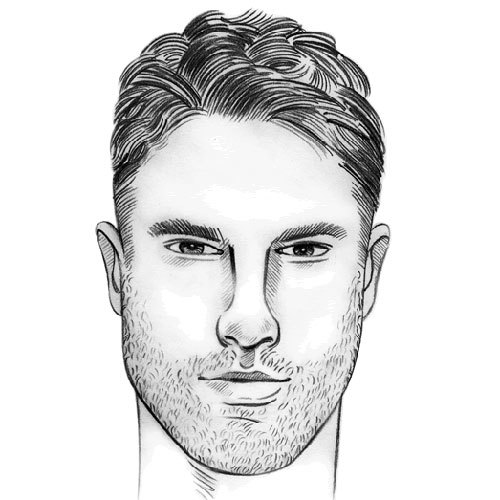Diamond菱形臉：C> B > A > D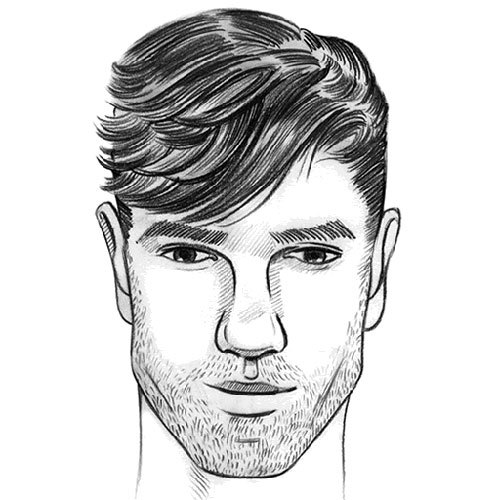Triangle三角形臉：D > B > A